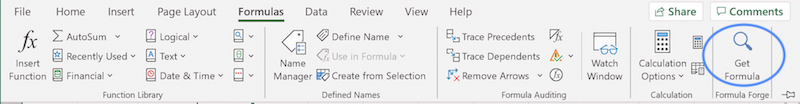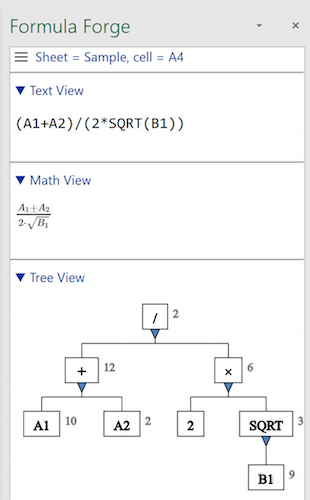# Introduction

Formula Forge helps users of Microsoft Excel® understand complicated formulas by presenting multiple views that clarify their meaning and structure. A forthcoming extension will let users create and modify formulas using intuitive drag-and-drop graphics instead of error-prone text entry.

• The text view shows the text of a formula, as it appears in Excel's formula bar.
• The math view shows a mathematically typeset, easier to read version of the formula.
• The tree view shows how Excel decomposes a formula into simpler elements.

Excel makes extensive use of parentheses, both to surround function arguments and to constrain the evaluation order. Without parentheses in a formula like `(A1+A2)/(2*SQRT(B1))`, Excel would not know to compute the numerator `A1+A2` and the denominator `2*SQRT(B1)` before it computed their quotient. However, so many parentheses in a formula can make it hard to read.

Formula Forge adds a `Get Formula` buttons to Excel's `Formulas` ribbon.New button on Excel's `Formulas` ribbon

Clicking the `Get Formula` button causes Formula Forge to show its math and tree views, uncluttered by parentheses, in addition to the text view for the formula in the selected cell.Three views of a simple formula

Users can select an element in a formula for attention by clicking it in any of the three views. They can also collapse and expand elements to suppress or reveal detail. Formula Forge synchronizes all three views so that whatever the user does in any one view is reflected automatically in the others.